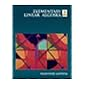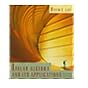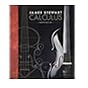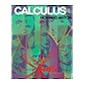Normal view

# Calculus / James Stewart.

Material type:TextLanguage: English Publication details: c2016. Edition: 8th edDescription: 1 volume (various pagings) : illustrations (some color) ; 26 cmISBN: 9781285740621 (student edition); 1285740629 (student edition); 9781305271760 (looseleaf edition); 1305271769 (looseleaf edition)Subject(s): Calculus -- Textbooks | CalculusGenre/Form: Textbooks. DDC classification: 515 LOC classification: QA303.2 | .S73 2016Online resources: Ebook Fulltext
Contents:
Functions and limits -- Derivatives -- Applications of differentiation -- Integrals -- Applications of integration -- Inverse functions -- Techniques of integration -- Further applications of integration -- Differential equations -- Parametric equations and polar coordinates -- Infinite sequences and series -- Vectors and the geometry of space -- Vector functions -- Partial derivatives -- Multiple integrals -- Vector calculus -- Second-order differential equations.
Tags from this library: No tags from this library for this title.
Star ratingsAverage rating: 0.0 (0 votes)
Holdings
Item type Current library Collection Call number Status Date due Barcode Item holdsE-Book
Circulation Section
Non-fiction 515 (Browse shelf(Opens below)) Not for loan
Total holds: 0
##### Browsing EWU Library shelves, Shelving location: Circulation Section Close shelf browser (Hides shelf browser)512 ANE 1994 Elementary linear algebra / 512 HET 2002 Topics in algebra / 512 LAL 1996 Linear algebra and its applications / 515 Calculus / 515.15 ANC 1995 Calculus with analytic geometry / 515.15 ANC 1995 Calculus with analytic geometry / 515.15 ANC 1999 Calculus :

Includes index.

Functions and limits -- Derivatives -- Applications of differentiation -- Integrals -- Applications of integration -- Inverse functions -- Techniques of integration -- Further applications of integration -- Differential equations -- Parametric equations and polar coordinates -- Infinite sequences and series -- Vectors and the geometry of space -- Vector functions -- Partial derivatives -- Multiple integrals -- Vector calculus -- Second-order differential equations.

MPS

Abdul Latif

There are no comments on this title.